# Catch up on the latest AI articles# 【Lambda Networks】No Need For Attention! This Is The New Generation Of Lambda Networks!

3 main points
✔️ LambdaNetworks, an alternative to Attention, is proposed and adopted in ICLR2021
✔️ Consider a wide range of interactions without using Attention
✔️ Outperforms Attention and Convolution models in terms of both computational efficiency and accuracy

written by Irwan Bello
(Submitted on 17 Feb 2021)
Comments: Accepted by the International Conference in Learning Representations 2021 (Spotlight)

Subjects: Computer Vision and Pattern Recognition (cs.CV); Machine Learning (cs.LG)code：The images used in this article are either from the paper or created based on it.

## First of all

Modeling long-term dependencies in data has been studied as a central problem in machine learning, and Self-Attention has recently gained attention as a popular approach to do so, but it is very expensive to compute and has not been applied to multidimensional data such as long sequences and images. The Linear Attention mechanism proposes a scalable solution to this high memory requirement, but it fails to model internal data structures such as relative distances between pixels or edge relationships between nodes in a graph.

Therefore, the LambdaNetworks proposed in this research solves these two problems. We propose LambdaLayer, which models the long-range interaction between a Query and a structured Context element with low memory cost. While Self-Attention defines a similarity kernel between a Query and a Context element, Lambda Layer summarizes Context information into a fixed-size linear function (i.e., matrix), thus avoiding the need for a memory-intensive attention map. It avoids the need for memory-hungry attention maps.

As shown in the experimental results below, LambdaNetworks significantly outperforms Convolution- and Attention-based models in terms of accuracy, and at the same time, it is computationally efficient and fast. This Lambda Layer can be easily implemented with einsum operations and convolutional kernels, and is available on Github. It is also known that the performance can be improved by simply replacing a part of the existing model such as ResNet with Lambda Layer.

## Lambda Layer Description

### overall image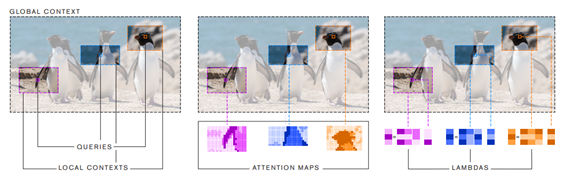Capturing the interaction over a long distance is the same as the problem of considering the Global Context in the above figure. The problem is that the usual Convolution can only consider the small kernel size part and cannot consider the Global Context. Therefore, Attention, which is often used nowadays, considers the Global Context by introducing the Attention Map, which calculates the importance of each pixel and all other pixels. Note that the Local Context shows only a part of the image in the above figure, but in Self-Attention and Lambda, the Local Context is often extended to the whole image, i.e., the same size as the Global Context. Now, the Attention Map can take into account the Global Context, but at the same time, it is known to be very computing expensive because it needs to compute a different Attention Map for the whole image for every single pixel. Therefore, as you can see in the above figure, Lambda aggregates the information more abstractly than the Attention Map, and then asks Lambda for the Global Context only once. The details will be described later, but I hope you have a rough idea of the difference between Attention and Lambda.

### attention

In order to explain Lambda, it is easiest to compare the difference between Lambda and Attention, so I would like to review Attention first.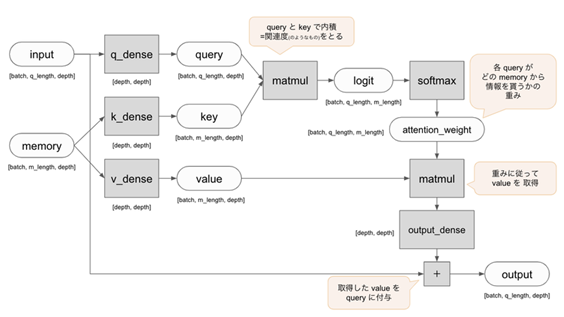The above diagram sums up Attention so succinctly that it needs no further explanation. Here, memory is used twice in the form of key and value, where key is used for searching and value is used as the value itself, and this mechanism is used in the same way in Lambda (the word memory is called context in Lambda ). In Self-Attention, input and memory have the same content, but in Lambda, input and context often have the same content as well. the key point in Attention is to take the inner product of query and key, and if the vectors are similar, the value will be larger. The key point of Attention is that the relevance is calculated by taking the inner product of query and key, using the property that the value becomes larger if the vectors are similar, and then nonlinearized by softmax to obtain the Attention Map, which is output by taking the product with value. This can be expressed as follows.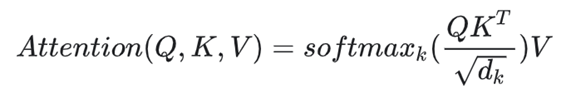The above flow of Attention can be rewritten to explain Lambda as shown in the figure below. Note that context is the same meaning as memory.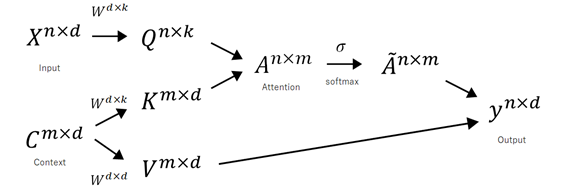### Lambda Layer

This is a long preamble, but I will now finally explain the Lambda Layer. First of all, the figure below illustrates the same as in the case of Attention.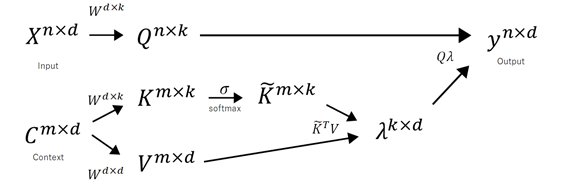You can easily see the difference by comparing with the Attention figure. The difference is that the softmax is not taken on the inner product of Query and Key, but only on Key. According to the author, what is meant by nonlinearizing the Key with softmax and taking the product of the Key and the value is that the content is summarized. Recall the image of Lambda that I described before, which summarizes information in a more abstract way than Attention. In fact, by converting from Context to λ, the dimensionality is reduced from m to k, and you can see that it works like a dimensionality reduction. You can see that this architecture is based on Attention, but it is completely different from Attention.

### position lambda

The lambda described above is called content lambda in the paper. As you can see, the content lambda can summarize the context, but it cannot capture the positional relationship in the image. Therefore, the position lambda was introduced together with the content lambda to take into account the relative positional relationships.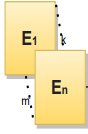E will be an indexed tensor of dimension N×M×K. They are a fixed set of learned parameters and serve as positional embeddings. However, in practice, the embedding is located directly on the input, so the size is M × K, and it is multiplied by N inputs. The key point is that this N×M and K-dimensional vector matrix is learned layer by layer, and the computation is not changed from example to example. Therefore, N×M×K is fixed in memory and does not grow with the batch size.

### whole picture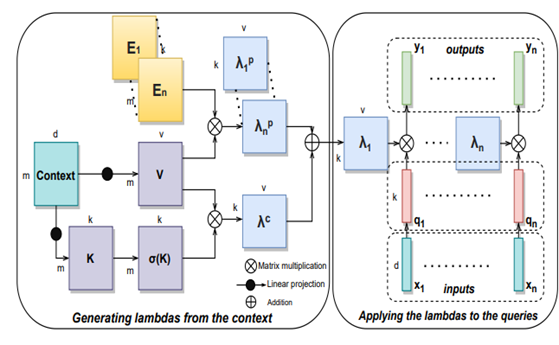The above figure shows the content lambda, the position lambda, and finally the product of Query and λ. Then, the equation is as follows. Based on the previous explanations, I think there is no problem.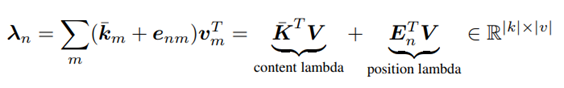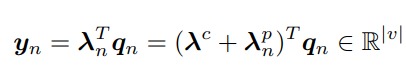## implementation code

The Lambda Layer can be found on Github (https://github.com/lucidrains/lambda-networks ), and you can find a brief description of the code in the paper.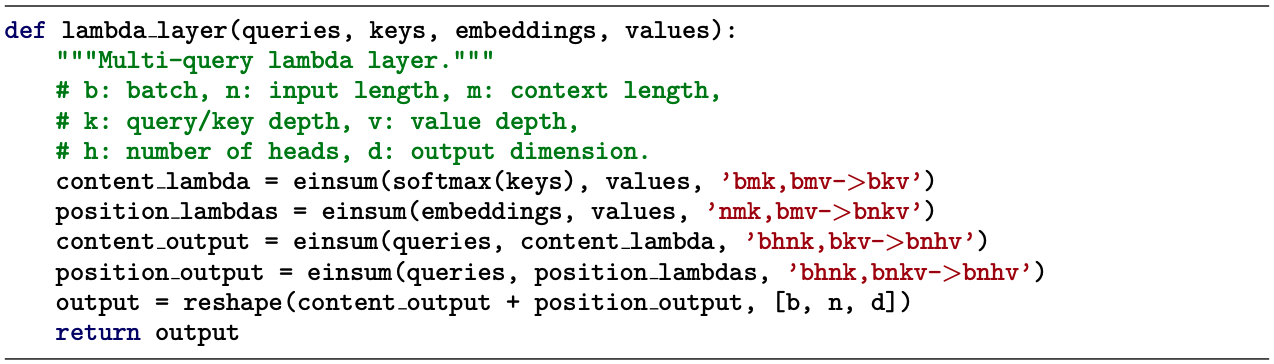You can see from the above code that it is very easy to define a Lambda Layer. Here, einsum is a built-in function in pytorch that allows you to perform multi-dimensional linear algebraic array operations in a simplified form, based on Einstein's contraction notation. However, using einsum, you can easily perform tensor product operations.

The Lambda Layer is designed to be very simple, so it is easy to rewrite only a part of an existing model, such as ResNet, into a lambda layer. The LambdaResNet mentioned in the paper is also available as a PyTorch implementation on Github by a volunteer (https://github.com/leaderj1001/LambdaNetworks ).

## experimental results

The author compares Lambda Layer with convolutional networks and Self-Attention.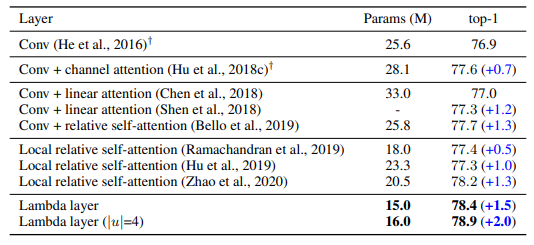As can be seen from the above figure, the number of parameters of Lambda Layer is very small compared to other methods, only 15 or 16M. However, it achieves better accuracy than other methods in the ImageNet classification task.

The figure below also shows the memory cost and accuracy, and you can see that Lambda Layer achieves high accuracy with very little memory, especially compared to Self-Attention.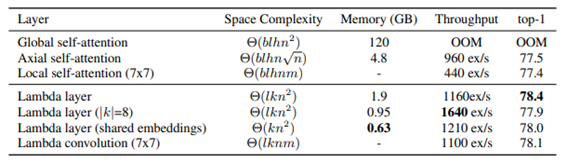The figure below also illustrates the tradeoff between accuracy and training time. Again, it is shown that LambdaResNet achieves higher accuracy with less training time than EfficientNet.